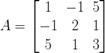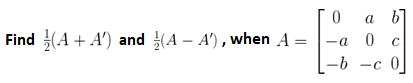NCERT Solutions for Maths Class 12 Exercise 3.3

# NCERT Solutions for Maths Class 12 Exercise 3.3

## NCERT Solutions for Maths Class 12 Exercise 3.3

### Maths Class 12 Ex 3.3 Question 1.

Find the transpose of each of the following matrices:Solution:

Solution:

### Maths Class 12 Ex 3.3 Question 7.

(i) Show that the matrixis a symmetric matrix.

(ii) Show that the matrixis a skew-symmetric matrix.

Solution:
(i) For a symmetric matrix aij = aji
Now,Here, a12 = –1 = a21, a13 = 5 = a31, a23 = 1 = a32

Again, a11, a22, a33 are 1, 2, 3, respectively.

Hence, aij = aji

Therefore, A is a symmetric matrix.

### Maths Class 12 Ex 3.3 Question 8.

For the matrix,, verify that

(i) (A + A’) is a symmetric matrix.
(ii) (A – A’) is a skew-symmetric matrix.

Solution:

We have,

### Maths Class 12 Ex 3.3 Question 9.Solution:

We have,

### Maths Class 12 Ex 3.3 Question 10.

Express the following matrices as the sum of a symmetric and a skew-symmetric matrix.Solution:

Choose the correct answer in the following questions:

### Maths Class 12 Ex 3.3 Question 11.

If A, B are symmetric matrices of same order, then AB – BA is a
(A) Skew symmetric matrix
(B) Symmetric matrix
(C) Zero matrix
(D) Identity matrix

Solution:
Since A and B are symmetric matrices, A’ = A and B’ = B.
Then, (AB – BA)’ = (AB)’ – (BA)’
= B’A’ – A’B’
= BA – AB
= –(AB – BA)
Therefore, AB – BA is a skew-symmetric matrix.

Hence, option (A) is correct.

### Maths Class 12 Ex 3.3 Question 12.

If, then A + A’ = I, if the value of α is

(A) π/6            (B) π/3             (C) π             (D) 3π/2

Solution:
We have,

Hence, option (B) is correct.